## General Question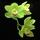# Algebra help? Find the domain of...

Asked by MyNewtBoobs (19036) October 3rd, 2010

Find the domain of:
f(x)=(10-x)/√(3x+5)

I don’t even know where to start. How do I go about solving this? Or finding the domain or whatever?

Observing members: 0Composing members: 0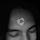The domain is the range of values of x for which f(x) can be evaluated.

Things that are not in the domain are values of x for which (10-x)/√(3x+5) is not a number—for instance if calculating it involves dividing by zero, then it’s not a number. Or if it involves imaginary numbers (i.e., square rooting a negative number) for your purposes it’s probably not in the domain.

lapilofu (4325)“Great Answer” (2) Flag as…@lapilofu So then how do I go about solving it, other than plain guessing?

MyNewtBoobs (19036)“Great Answer” (0) Flag as…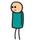You can check out the explanation given here.

bob_ (19690)“Great Answer” (1) Flag as…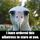@papayalily I’d suggest studying, for starters. Every function has some constraint it has to respect, the object of even roots has to be >0, and you can’t divide by 0. These are two starters, if you still don’t know maybe you should read up on a subject before starting to do your homework.

Also writing f(x)= is exactly like writing y=

Thammuz (9272)“Great Answer” (1) Flag as…@Thammuz There’s no need to get snarky with me. I did study trying to understand it for 2 hours. I’m in the exact same place. That’s why I came here.

MyNewtBoobs (19036)“Great Answer” (1) Flag as…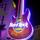@papayalily I dont have a better answer for you as far as solving the problem goes.

However, as someone who absolutely hates/can’t understand algebra, I’m with you when I say – if people would just explain the material properly, carefully and articulately, the subject would be a lot easier.

I have a suggestion – find a good tutor. It helped me wonders. If that’s not an option, go to your professor after class.

Disc2021 (4488)“Great Answer” (1) Flag as…@Disc2021 I ask questions during class. He doesn’t answer them. I mean, he answers a question, but not the question. Last class, one student asked if what he had written was an x (because his handwriting is illegible) and he spent 15 minutes going back over the formula so that she would understand why it was x, but never actually confirmed that it was an x, and not a 4 or a y….

MyNewtBoobs (19036)“Great Answer” (0) Flag as…@papayalily To find the domain you have to find the values of X that satisfy the two conditions i have specified: you have a fraction and a root, you have to verify what values allow for a result from them. So find what values make the part inside the root >=0 and then exclude the value that makes it equal to zero, because you can’t divide by zero, and you’re good to go.

As for solving it, there is nothing to solve, it’s a function.

Thammuz (9272)“Great Answer” (0) Flag as…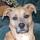Wolfram Alpha can help a lot. Just seeing the function graphed can work wonders. It’s a great website. It won’t help to show your work so much as it will help to show what your work should look like.

CyanoticWasp (20070)“Great Answer” (0) Flag as…First thing to realize is that the numerator is not going to cause difficulties. Any value of x will work in the numerator. The thing to check then is the denominator. You know that the value under the square root sign must be greater than or equal to 0 in order to be able to take a square root. You also know that it can not be zero because you can’t have 0 in the denominator. So the condition is that the expression under the radical must be greater than 0. You can then work with that expression to isolate x and thus come up with the range of values of x. That is the best I can do short of doing the problem for you.

LostInParadise (26919)“Great Answer” (0) Flag as…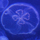f(x)=(10-x)/√(3x+5) , f(x): x =/= -5/3

where =/= means cannot

I figured it out by looking at √(3x+5). I thought to myself, what can make it zero. There is also an addition sign there, so x must be negative to achieve 0. Also, since it is a 3 and 5 is not divisible by 3, it must be a fraction.

so i thought, what plus 5 can equal zero? negative 5 plus 5 can equal zero. so, i thought, what can be multiplied by 3 to make zero? 3/5.

just a mode of thinking if you’re interested beyond an answer. there ARE tests you know.

gravityalwaysbringsmedown (70)“Great Answer” (1) Flag as…or